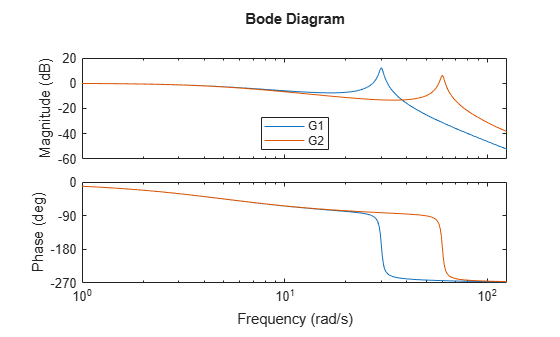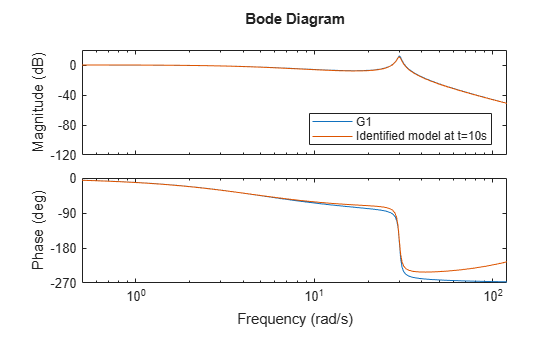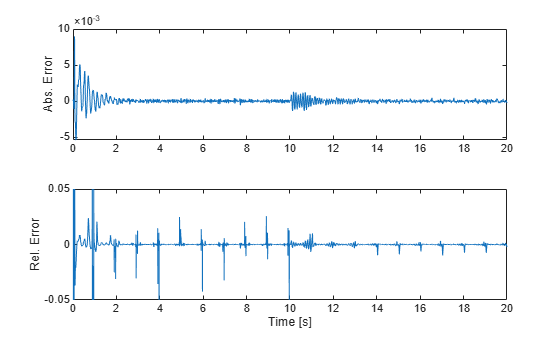## Online ARX Parameter Estimation for Tracking Time-Varying System Dynamics

This example shows how to perform online parameter estimation for a time-varying ARX model at the MATLAB command line. The model parameters are updated at each time step with incoming new data. This model captures the time-varying dynamics of a linear plant.

### Plant

The plant can be represented as:

`$y\left(s\right)=G\left(s\right)u\left(s\right)+e\left(s\right)$`

Here, G is the transfer function and e is the white-noise. The plant has two operating modes. In the first operating mode, the transfer function is:

`$G1\left(s\right)=\frac{4500}{\left(s+5\right)\left({s}^{2}+1.2s+900\right)}$`

The lightly damped poles in G1(s) have the damping 0.02 and natural frequency 30rad/s. In the second operating mode, the natural frequency of these poles is 60rad/s. In this mode, the transfer function is:

`$G2\left(s\right)=\frac{18000}{\left(s+5\right)\left({s}^{2}+2.4s+3600\right)}$`

The plant operates in the first mode until t=10s, and then switches to the second mode.

The Bode plots of G1 and G2 are:

```wn = 30; % natural frequency of the lightly damped poles ksi = 0.02; % damping of the poles G1 = tf(1,conv([1/5 1],[1/wn^2 2*ksi/wn 1])); % plant in mode 1 wn = 60; % natural frequency in the second operating mode G2 = tf(1,conv([1/5 1],[1/wn^2 2*ksi/wn 1])); % plant in mode 2 bode(G1,G2,{1,125}); legend('G1','G2','Location','Best');```### Online ARX Parameter Estimation

The aim is to estimate the dynamics of the plant during its operation. ARX is a common model structure used for this purpose. ARX models have the form:

`$y\left(t\right)=\frac{B\left(q\right)}{A\left(q\right)}u\left(t\right)+\frac{1}{A\left(q\right)}e\left(t\right)$`

Here, ${q}^{-1}$ is the time-shift operator. The ratio of the polynomials B(q)/A(q) captures the input-output model (u(t) to y(t)), and 1/A(q) captures the noise model (e(t) to y(t)). You are estimating the coefficients of the A(q) and B(q) polynomials. e(t) is white noise.

ARX model structure is a good first candidate for estimating linear models. The related estimation methods have low computational burden, are numerically robust, and have the convexity property. The convexity property ensures there is no risk of parameter estimation getting stuck at a local optima. However, ARX model structure does not provide flexibility for noise models.

The lack of flexibility in noise modeling can pose difficulties if the structure of the plant does not match with the ARX model structure, or if the noise is not white. Two approaches to remedy this issue are:

1. Data Filtering: If the noise model is not important for your application, you can use data filtering techniques. For more details, see the 'Filter the Data' section.

2. Different model structures: Use ARMAX, Output-Error, and Box-Jenkins polynomial models for more flexibility in model structures.

### Select Sample Time

The sample time choice is important for good approximation of the continuous-time plant by a discrete-time model. A rule of thumb is to choose the sampling frequency as 20 times the dominant dynamics of the system. The plant has the fastest dominant dynamics at 60rad/s, or about 10Hz. The sampling frequency is therefore 200Hz.

`Ts = 0.005; % [s], Sample time, Ts=1/200`

### System Excitation

For successful estimation the plant inputs must persistently excite its dynamics. Simple inputs such as a single step input are typically not sufficient. In this example the plant is driven by a pulse with amplitude 10 and a period of 1 second. The pulse width is 50% of its period.

Generate plant input and output signals:

```t = 0:Ts:20; % Time vector u = double(rem(t/1,1)-0.5 < 0); % pulse y = zeros(size(u)); % Store random number generator's states for reproducible results. sRNG = rng; rng('default'); % Simulate the mode-switching plant with a zero-order hold. G1d = c2d(G1,Ts,'zoh'); B1 = G1d.num{1}.'; A1 = G1d.den{1}.'; % B1 and A1 corresponds to G1. G2d = c2d(G2,Ts,'zoh'); B2 = G2d.num{1}.'; A2 = G2d.den{1}.'; % B2 and A2 corresponds to G2. idx = numel(B2):-1:1; for ct=(1+numel(B2)):numel(t) idx = idx + 1; if t(ct)<10 % switch mode after t=10s y(ct) = u(idx)*B1-y(idx(2:end))*A1(2:end); else y(ct) = u(idx)*B2-y(idx(2:end))*A2(2:end); end end % Add measurement noise y = y + 0.02*randn(size(y)); % Restore the random number generator's states. rng(sRNG);```

Plot the input-output data:

```figure(); subplot(2,1,1); plot(t,u); ylabel('Input (u)'); subplot(2,1,2); plot(t,y); ylim([-0.2 1.2]) ylabel('Output (y)'); xlabel('Time [s]');```### Filter the Data

The plant has the form:

`$y\left(t\right)=G\left(q\right)u\left(t\right)+e\left(t\right)$`

where e(t) is the white noise. In contrast, the ARX models have the form

`$y\left(t\right)=\frac{B\left(q\right)}{A\left(q\right)}u\left(t\right)+\frac{1}{A\left(q\right)}e\left(t\right)$`

The estimator will use B(q) and A(q) to approximate G(q). However, note the difference in the noise models. The plant has white-noise e(t) directly impacting y(t), but the ARX model assumes that a white noise term filtered by 1/A(q) impacts y(t). This mismatch will negatively affect the estimation.

When the noise model is not of interest, one method to reduce the impact of this mismatch is to use a data filter. Use a filter $F\left(q\right)$ on both u(t) and y(t) to obtain ${u}_{f}\left(t\right)=F\left(q\right)u\left(t\right)$ and ${y}_{f}\left(t\right)=F\left(q\right)y\left(t\right)$. Then use the filtered signals ${u}_{f}\left(t\right)$ and ${y}_{f}\left(t\right)$ in the estimator instead of the plant input u(t) and output y(t). The choice of data filter lets you reduce the influence of e(t) on the estimation.

The data filter F(q) is typically a low-pass or a band-pass filter based on the frequency range of importance for the application, and the characteristics of e(t). Here, a 4th order Butterworth low-pass filter with cutoff frequency 10Hz is used. This is approximately the frequency of the fastest dominant dynamics in the plant (60rad/s). A low-pass filter is sufficient here because the noise term does not have low-frequency content.

```% Filter coefficients Fa = [1 -3.1806 3.8612 -2.1122 0.4383]; % denominator Fb = [4.1660e-04 1.6664e-03 2.4996e-03 1.6664e-03 4.1660e-04]; % numerator % Filter the plant input for estimation uf = filter(Fb,Fa,u); % Filter the plant output yf = filter(Fb,Fa,y);```

### Set Up the Estimation

Use the recursiveARX command for online parameter estimation. The command creates a System object™ for online parameter estimation of an ARX model structure. Specify the following properties of the object:

• Model orders: [3 1 0]. na = 3 because the plant has 3 poles. nk = 0 because plant does not have input delay. nb = 1 corresponds to no zeros in the system. nb was set after a few iterations, starting from nb=4 which corresponds to three zeros, and hence a proper model. A smaller number of estimated parameters are desirable and nb=1 yields sufficient results.

• EstimationMethod: 'ForgettingFactor' (default). This method has only one scalar parameter, ForgettingFactor, which requires limited prior information regarding parameter values.

• ForgettingFactor: 0.995. The forgetting factor, $\lambda$, is less than one as the parameters vary over time. $\frac{1}{1-\lambda }=200$ is the number of past data samples that influence the estimates most.

```X = recursiveARX([3 1 0]); % [na nb nk] X.ForgettingFactor = 0.995;```

Create arrays to store estimation results. These are useful for validating the algorithms.

```np = size(X.InitialParameterCovariance,1); PHat = zeros(numel(u),np,np); A = zeros(numel(u),numel(X.InitialA)); B = zeros(numel(u),numel(X.InitialB)); yHat = zeros(1,numel(u));```

Use the step command to update the parameter values using one set of input-output data at each time step. This illustrates the online operation of the estimator.

```for ct=1:numel(t) % Use the filtered output and input signals in the estimator [A(ct,:),B(ct,:),yHat(ct)] = step(X,yf(ct),uf(ct)); PHat(ct,:,:) = X.ParameterCovariance; end```

View the Bode plot of the estimated transfer functions:

```G1Hat = idpoly(A(1000,:),B(1000,:),1,1,1,[],Ts); % Model snapshot at t=10s G2Hat = idpoly(X); % Snapshot of the latest model, at t=20s G2Hat.Ts = G1d.Ts; % Set the sample time of the snapshot figure(); bode(G1,G1Hat); xlim([0.5 120]); legend('G1','Identified model at t=10s','Location','Best');``````figure(); bode(G2,G2Hat); xlim([0.5 120]); legend('G2','Identified model at t=20s','Location','Best');```### Validating the Estimated Model

Use the following techniques to validate the parameter estimation:

1. View output estimate, yhat(t): The third output argument of the step method is the one-step ahead prediction of the output yhat(t). This is based on current model parameters as well as current and past input-output measurements. The relative and absolute error between y(t) and yhat(t) are measures of the goodness of the fit.

2. View the parameter covariance estimate, Phat(t): This is available with the ForgettingFactor and KalmanFilter estimation methods. It is stored in the ParameterCovarianceMatrix property of the estimator. The diagonals of Phat(t) are the estimated variances of the parameters. It should be bounded, and the lower the better.

3. Simulate the estimated time-varying model: Use u(t) and the estimated parameters to simulate the model to obtain a simulated output, ysim(t). Then compare y(t) and ysim(t). This is a more strict validation than comparing y(t) and yhat(t) because ysim(t) is generated without the plant output measurements.

The absolute error yf(t)-yhat(t) and the relative error (yf(t)-yhat(t))/yf(t) are:

```figure(); subplot(2,1,1); plot(t,yf-yHat); ylabel('Abs. Error'); subplot(2,1,2); plot(t,(yf-yHat)./yf); ylim([-0.05 0.05]); ylabel('Rel. Error'); xlabel('Time [s]');```The absolute errors are on the order of 1e-3, which is small compared to the measured output signal itself. The relative error plot at the bottom confirms this, with errors being less than 5% except at the beginning of the simulation.

The diagonals of the parameter covariance matrix, scaled by the variance of the residuals y(t)-yhat(t), capture the variances of parameter estimates. The square-root of the variances are the standard deviations of the parameter estimates. The first three elements on the diagonals are the three parameters estimated in the A(q) polynomial. The last element is the single parameter in the B(q) polynomial. Let's look at the first estimated parameter in A(q)

```noiseVariance = var(yf-yHat); X.A(2) % The first estimated parameter. X.A(1) is fixed to 1```
```ans = -2.8635 ```
`sqrt(X.ParameterCovariance(1,1)*noiseVariance)`
```ans = 0.0175 ```

The standard deviation 0.0175 is small relative to the absolute value of the parameter value 2.86. This indicates good confidence in the estimated parameter.

```figure(); plot(t,sqrt(PHat(:,1,1)*noiseVariance)); ylabel('Standard deviation estimate for the parameter A(2)') xlabel('Time [s]');```The uncertainty is small and bounded throughout the estimation. However, note that the parameter standard deviations are also estimates. They are based on the assumption that the residuals y(t)-yhat(t) are white. This depends on the estimation method, its associated parameters, the structure of the estimated model, and the input signal u. Differences between the assumed and the actual model structure, lack of persistent input excitation, or unrealistic estimation method settings can lead to overly optimistic or pessimistic uncertainty estimates.

Lastly, simulate the estimated ARX model using the stored history of estimated parameters. This simulation can also be done simultaneously with the estimation loop for validation during online operation.

```ysim = zeros(size(y)); idx = numel(B2):-1:1; for ct=(1+numel(B2)):numel(t) idx = idx + 1; ysim(ct) = u(idx(1))*B(idx(1),:)-ysim(idx(2:end))*A(ct,2:end)'; end figure(); subplot(2,1,1); plot(t,y,t,ysim); ylabel('System Output'); legend('Measured','Estimated','Location','Best'); subplot(2,1,2); plot(t,y-ysim); ylim([-0.5 0.5]); ylabel('Error, y(t)-ysim(t)'); xlabel('Time [s]');```The error is large initially, but it settles to a smaller value around t=5s for the first operating mode. The large initial error can be reduced by providing the estimator an initial guess for the model parameters and initial parameter covariance. When the plant switches to the second mode, the errors grow initially but settle down as time goes on as well. This gives confidence that the estimated model parameters are good at capturing the model behavior for the given input signal.

### Summary

You performed online parameter estimation for an ARX model. This model captured the dynamics of a mode-switching plant. You validated the estimated model by looking at the error between estimated, simulated, measured system outputs as well as the parameter covariance estimates.

##### SupportGet trial now